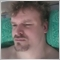Indicator testing issue (buffer indexing)24346

Hi,

I am having a bit of a problem here (again :)) with a simple test indicator (code below). When I test it in the strategy tester with all the arrays indexed from left to right the buffer values are as should and it also works fine on the chart. Then when I set all arrays indexed as timeseries it stops working properly. I.e. in the strategy tester values are not set into the indicator buffer and I must hit refresh to update the current bar value when the indicator is attached to a chart. The values are correct on the chart though. Please clarify this issue to me. Thanks.

Br, Candles

EDIT: Whoops :) noticed an error in my code :) Fix it now and see if it works then...

EDIT2: Nope :( Still the same problems...

//+------------------------------------------------------------------+
//|                                                        Range.mq5 |
//|                        Copyright 2013, MetaQuotes Software Corp. |
//|                                              http://www.mql5.com |
//+------------------------------------------------------------------+
#property copyright "Copyright 2013, MetaQuotes Software Corp."
#property version   "1.00"
#property indicator_chart_window
//---
#property indicator_buffers 1
//---
#property indicator_plots 1
//---
#property indicator_type1 DRAW_NONE
//---
#property indicator_style1 STYLE_SOLID
//---
#property indicator_label1 "Bull"

double bull_buff[];
//+------------------------------------------------------------------+
//| Custom indicator initialization function                         |
//+------------------------------------------------------------------+
int OnInit()
{
//--- indicator buffers mapping
ArraySetAsSeries(bull_buff,true);
SetIndexBuffer(0,bull_buff,INDICATOR_DATA);

PlotIndexSetDouble(0,PLOT_EMPTY_VALUE,EMPTY_VALUE);
//---
return(0);
}
//+------------------------------------------------------------------+
//| Custom indicator iteration function                              |
//+------------------------------------------------------------------+
int OnCalculate(const int rates_total,
const int prev_calculated,
const datetime &time[],
const double &open[],
const double &high[],
const double &low[],
const double &close[],
const long &tick_volume[],
const long &volume[],
{
//---
if(!ArraySetAsSeries(open,true))ArraySetAsSeries(open,true);
if(!ArraySetAsSeries(high,true))ArraySetAsSeries(high,true);
if(!ArraySetAsSeries(low,true))ArraySetAsSeries(low,true);
if(!ArraySetAsSeries(close,true))ArraySetAsSeries(close,true);
if(!ArraySetAsSeries(time,true))ArraySetAsSeries(time,true);

int FirstBar=0,i;

if(prev_calculated==0)FirstBar=1;
if(prev_calculated!=0)FirstBar=prev_calculated-1;

for(i=FirstBar;i<rates_total-1;i++)
{
bull_buff[i]=0;
if(open[i]<close[i])bull_buff[i]=1;
//if(open[i]>close[i])bull_buff[i]=0;
}
bull_buff[i]=0;

//--- return value of prev_calculated for next call
return(rates_total-1);
}
//+------------------------------------------------------------------+24346

After some fine debugging and strategy tester running I got it :) The solution (code below) was simple now the indicator works perfectly when the arrays are timeseries. It plots values to previous historical indicator buffer indexes plus updates the buffer index number 1 correctly when new bar forms. It also leaves the  buffer index 0 i.e. the current forming bar empty until the bar is complete. I think I have a better grasp of how accessing data and their ordering works :) One AHA moment was when I realized that there is no need for a for loop when setting buffer values for bars to come because OnCalculate() does just that.

//+------------------------------------------------------------------+
//|                                                        Simple.mq5 |
//|                        Copyright 2013, MetaQuotes Software Corp. |
//|                                              http://www.mql5.com |
//+------------------------------------------------------------------+
#property copyright "Copyright 2013, MetaQuotes Software Corp."
#property version   "1.00"
#property indicator_chart_window
//---
#property indicator_buffers 1
//---
#property indicator_plots 1
//---
#property indicator_type1 DRAW_NONE
//---
#property indicator_style1 STYLE_SOLID
//---
#property indicator_label1 "Bull"

double bull_buff[];
//+------------------------------------------------------------------+
//| Custom indicator initialization function                         |
//+------------------------------------------------------------------+
int OnInit()
{
//--- indicator buffers mapping
ArraySetAsSeries(bull_buff,true);

SetIndexBuffer(0,bull_buff,INDICATOR_DATA);

PlotIndexSetDouble(0,PLOT_EMPTY_VALUE,0);
//---
return(0);
}
//+------------------------------------------------------------------+
//| Custom indicator iteration function                              |
//+------------------------------------------------------------------+
int OnCalculate(const int rates_total,
const int prev_calculated,
const datetime &time[],
const double &open[],
const double &high[],
const double &low[],
const double &close[],
const long &tick_volume[],
const long &volume[],
{
//---
if(!ArraySetAsSeries(open,true))ArraySetAsSeries(open,true);
if(!ArraySetAsSeries(high,true))ArraySetAsSeries(high,true);
if(!ArraySetAsSeries(low,true))ArraySetAsSeries(low,true);
if(!ArraySetAsSeries(close,true))ArraySetAsSeries(close,true);
if(!ArraySetAsSeries(time,true))ArraySetAsSeries(time,true);

int FirstBar=0;

if(prev_calculated==0)FirstBar=1;
else FirstBar=prev_calculated-1;

for(int i=FirstBar;i<rates_total;i++)
{
bull_buff[i]=0.0;
if(close[i]>open[i])bull_buff[i]=1;
}
if(!bull_buff==0)bull_buff=0;
if(close>open)bull_buff=1;
//--- return value of prev_calculated for next call
return(rates_total);
}
//+------------------------------------------------------------------+